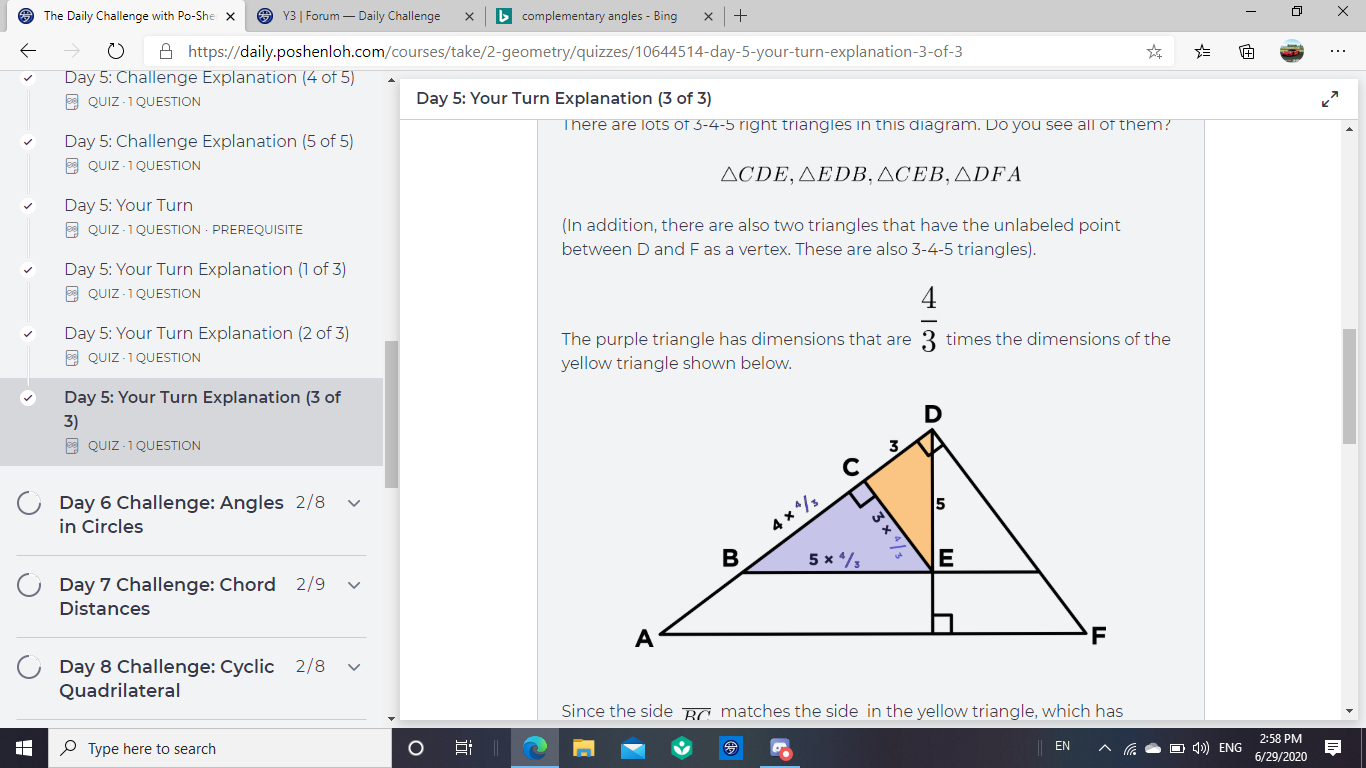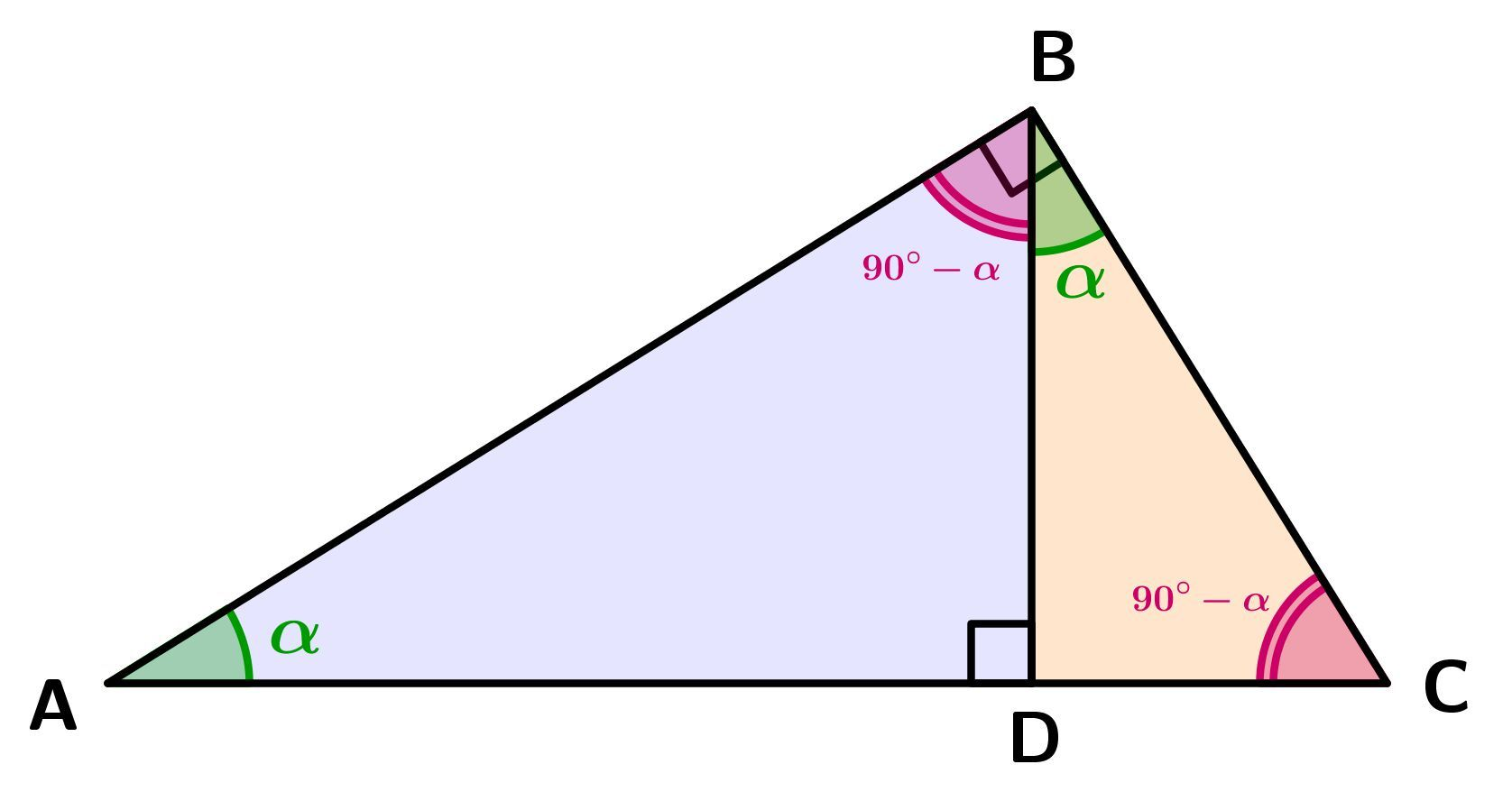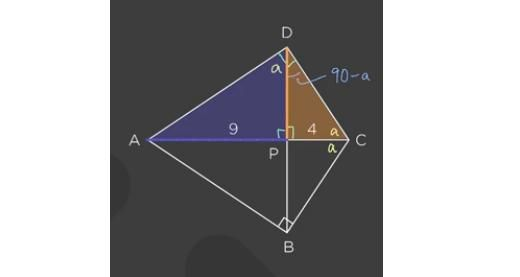# How do we know for sure these triangles are 3-4-5?

• I found a maybe-error here. (Probably not)How do we know for sure these triangles are 3-4-5? They sure look like it, but I thought we shouldn't believe in the pictures so much.

I may be repeating myself (maybe I did a post on this before), but at 4:00, why does 4/PD = PD/9?

• Module 2 Week 2 Day 5 Your Turn Explanation Part 3 Mini-Question

Nice question! Here you have to remember the following fact:

If you draw the height of a triangle, this divides the triangle into two right triangles, each of which is similar to the original one.Proof:
Let $$\angle BAC=\angle BAD={\color{green}\alpha}.$$ Then $$\angle BCD=\angle BCA=180^{\circ}-\angle ABC-\angle BAC={\color{purple}90^{\circ}-\alpha} \Rightarrow \angle DBC=180^{\circ}-\angle BDC-\angle BCD=180^{\circ}-90^{\circ}-({\color{purple}90^{\circ}-\alpha})={\color{green}\alpha}=\angle BAC=\angle BAD.$$ The same way we get that $$\angle ABD={\color{purple}90^{\circ}-\alpha}=\angle BCD=\angle BCA.$$ All this means that $$\bf \triangle ABC\sim {\color{blue}\triangle ADB}\sim {\color{darkorange}\triangle BDC}.$$ $$\boxed{}$$

$$CE$$ is a height in right $$\triangle BDE,$$ so $$\color{darkorange}\triangle CDE$$ and $$\color{purple}\triangle BCE$$ are similar to $$\triangle BDE.$$

The same thing works with the other triangles:
Let $$DE\cap AF=H$$ and $$BE\cap DF=K.$$ Then $$DH$$ is a height in right $$\triangle ADF,$$ so $$\triangle AHD$$ and $$\triangle DHF$$ are similar to $$\triangle ADF.$$

In addition, $$BE \parallel AH \Rightarrow \triangle BDE\sim \triangle ADH,$$ as well as $$EK \parallel HF \Rightarrow \triangle KDE\sim \triangle FDH.$$

So if one of these triangles is 3-4-5 right triangle, then all the others will also be 3-4-5 right triangles.The same fact that I mentioned before is used at 4:00 in order to get that equality:$$DP$$ is a height in right $$\triangle ADC,$$ so $$\triangle APD$$ and $$\triangle DPC$$ are similar to $$\triangle ADC,$$ which means that:$$\triangle APD\sim \triangle DPC \Rightarrow$$ $$\Rightarrow \frac{\color{darkorange}PC}{PD}=\text{ratio of similarity}=\frac{PD}{\color{blue}PA}$$ $$\Rightarrow \frac{\color{darkorange}4}{PD}=\frac{PD}{\color{blue}9}$$